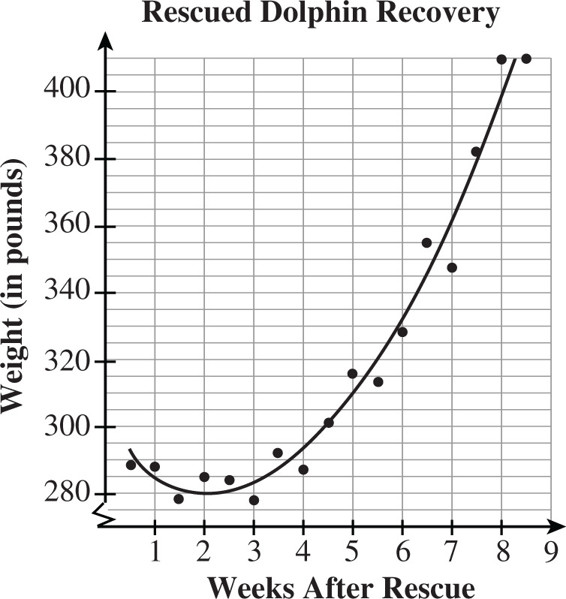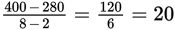# SAT Math Multiple Choice Question 506: Answer and Explanation

### Test Information

Question: 506

11.Following the catastrophic oil spill in the Gulf of Mexico in April of 2010, more than 900 bottlenose dolphins were found dead or stranded in the oil spill area. The figure above shows the weight of a rescued dolphin during its recovery. Based on the quadratic model fit to the data shown, which of the following is the closest to the average rate of change in the dolphin's weight between week 2 and week 8 of its recovery?

• A. 4 pounds per week
• B. 16 pounds per week
• C. 20 pounds per week
• D. 40 pounds per week

Explanation:

C

Difficulty: Medium

Category: Problem Solving and Data Analysis / Scatterplots

Strategic Advice: The average rate of change of a function over a given interval, from a to b, compares the change in the outputs, f(b) - f(a), to the change in the inputs, b - a. In other words, it is the slope of the line that connects the endpoints of the interval, so you can use the slope formula.

Getting to the Answer: Look at the quadratic model, not the data points, to find that the endpoints of the given interval, week 2 to week 8, are (2, 280) and (8, 400). The average rate of change is.

On average, the dolphin's weight increased by 20 pounds per week.